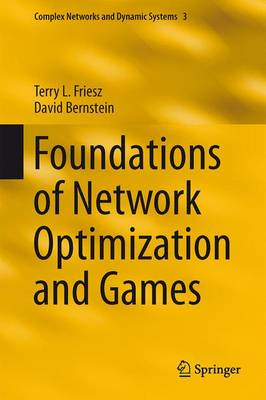•# Foundations of Network Optimization and Games - Complex Networks and Dynamic Systems 3 (Hardback)

(author), (author)
£129.99
Hardback 504 Pages / Published: 01/10/2015
• We can order this

Usually dispatched within 3 weeks

This is a book about infrastructure networks that are intrinsically nonlinear. The networks considered range from vehicular networks to electric power networks to data networks.

The main point of view taken is that of mathematical programming in concert with finite-dimensional variational inequality theory. The principle modeling perspectives are network optimization, the theory of Nash games, and mathematical programming with equilibrium constraints. Computational methods and novel mathematical formulations are emphasized. Among the numerical methods explored are network simplex, gradient projection, fixed-point, gap function, Lagrangian relaxation, Dantzig-Wolfe decomposition, simplicial decomposition, and computational intelligence algorithms.

Many solved example problems are included that range from simple to quite challenging. Theoretical analyses of several models and algorithms, to uncover existence, uniqueness and convergence properties, are undertaken. The book is meant for use in advanced undergraduate as well as doctoral courses taught in civil engineering, industrial engineering, systems engineering, and operations research degree programs. At the same time, the book should be a useful resource for industrial and university researchers engaged in the mathematical modeling and numerical analyses of infrastructure networks.

Publisher: Springer-Verlag New York Inc.
ISBN: 9781489975935
Number of pages: 504
Weight: 9927 g
Dimensions: 235 x 155 x 33 mm
Edition: 1st ed. 2016

MEDIA REVIEWS

"This book is about nonlinear deterministic network models. ... Each chapter is self-contained and has its own reference list. The book's main aim is to present a tool kit to model and solve complex network problems in practice. ... It is easy to follow the exposition. ... this book provides a good guideline for first and second reading." (Mechthild Opperud, Mathematical Reviews, June, 2016)

"The book presents an excellent comprehensive introduction to the area of network flow problems, where not only a system optimum is searched, but also the behaviour of competing non-cooperative users is taken into account. ... Generally, it can be stated that the book constitutes a precious guide for researchers and advanced students to the field of network flow problems." (Jaroslav Janacek, zbMATH 1344.90062, 2016)